Courses

# Polytropic Process, Entropy Change and Spontaneity Class 11 Notes | EduRev

## Class 11 : Polytropic Process, Entropy Change and Spontaneity Class 11 Notes | EduRev

The document Polytropic Process, Entropy Change and Spontaneity Class 11 Notes | EduRev is a part of the Class 11 Course Chemistry for JEE.
All you need of Class 11 at this link: Class 11

POLYTROPIC PROCESS
PVx = Const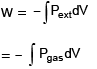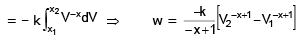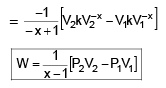MOLAR HEAT CAPACITY OF POLYTROPIC PROCESS
dU = dq + dW
nCVdT = nCmdT (-PdV)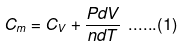PV = nRT
KV-x V = nRT
kV-x 1 = nRT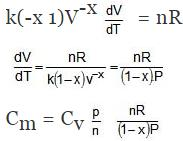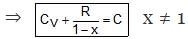Limitations of First law of Thermodynamics
(a) First law of thermodynamics does not give information regarding the direction of propagation of a process
(b) First law of thermodynamics does not tell us why an equilibrium is attained.
(c) First law of thermodynamics does not tell us when an equilibrium will be attained.
(d) First law of thermodynamics does not give information about why there can not be 100 percent conversion of heat into work

Second Law of Thermodynamics
Statement(I) : Second law of thermodynamics states that heat can never be converted into work with 100% efficiency
Statement(II) : No engine in this world can be constructed which operates in cycles and converts all the heat from source to work.
Statement(III) : No refrigertator can be designed which operates in cycles and rejects heat from sink to source, perpetually (self - functioning).
Entropy : Entropy is the direct measurment of randomness or disorderness. Entropy is an extensive property & it is a state function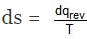for reversible process. entropy is related with complexity of the molecule within the system.
EtOH > MeOH
C2H6 (g) > C2H5(g)
N2O4 > NO2
O2 > N2 (molecular wt.)
Gas > Liq > Amorphous solid > crystalline solid

Entropy always increases in the following process
(a) s → ℓ, ℓ → g, s → g,
(b) Isothermal expansion of ideal gas.
(c) Mixing of two non reacting gases.
(d) In chemical reaction in which

Δng > 0
(e) Heating of any substance

Classification of process Based on spontaneity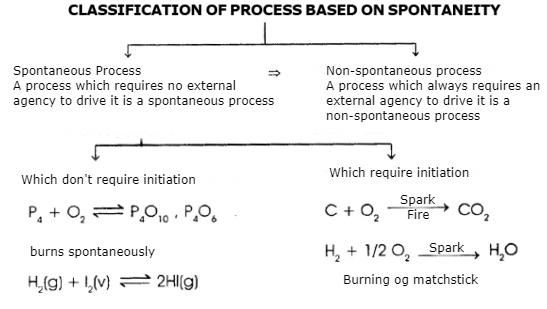Why Spontaneity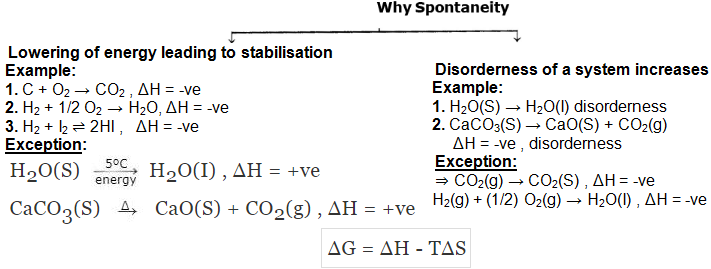Points to ponder:
Why a system always moves towards disorderness ?
Answer: A system moves towards disorderness because the probability of moving towards disorderness is very high.

DISORDERNESS

For Reversible process: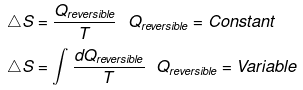Note : Irreversible process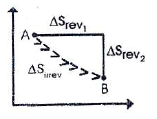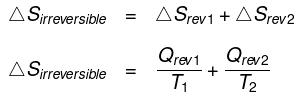The entropy change for an irreversible process can be calculated by substituting it with equivalent reversible process. Both will have same entropy change.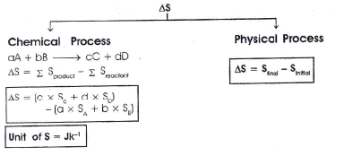Entropy Change of Universe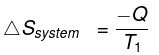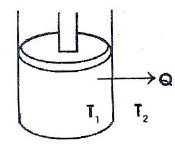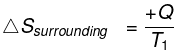ΔSuniverse = ΔSsystem ΔSsurrounding

Spontaneity

1. ΔSuniverse > 0 ⇒ Spontaneous

2. ΔSuniverse = 0 ⇒ Equilibrium

3. ΔSuniverse < 0 ⇒ Non-spontaneous.

Entropy Change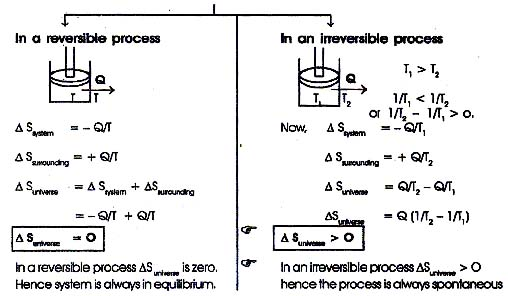Calculation of entropy change

(A) General heating or cooling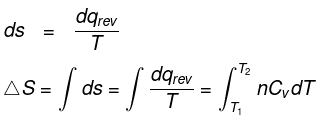If C is temperature independent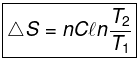If C is a function of Temperature

C = a + bT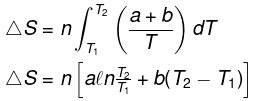(B) In phase transformation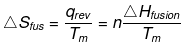*A(l)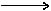A(g).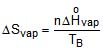*A(s)A(g)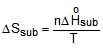(C) Entropy change during chemical reaction.

aA bB → cC dD
ΔSº = entropy of product - entropy of reac tan t

For any chemical reaction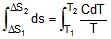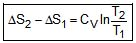(D) Calculation of entropy change during expansion/compression of ideal gas from P1V1T1 to P2V2T2

From Ist law of thermodynamics

dE = dq + dW

dq = - dW + dE

TdS = PdV + nCVdT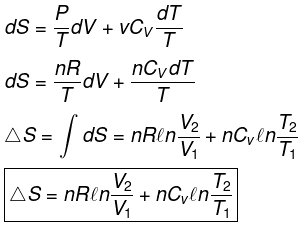For ideal gas

ΔS = nCVln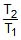+ nRln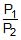.= nCVlnnRlnnRln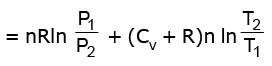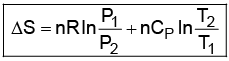Calculation of entropy change during isothermal Expansion;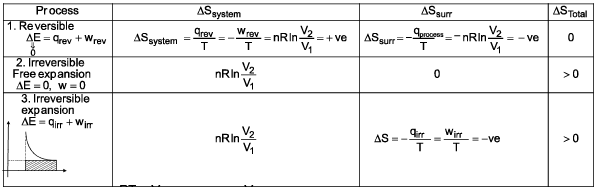because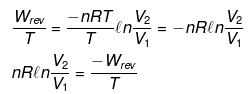Wrev > Wirr

ΔStotal = +ve

Calculation of entropy change during isothermal compression.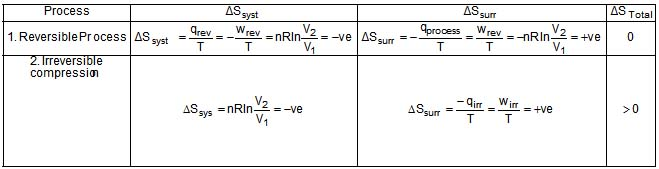qirr + wirr = 0

| wirr | > | wrev |

< 0 >0

⇒ | qirr | > | qrev |

Calculation of entropy change during for adiabatic expansion of ideal gas.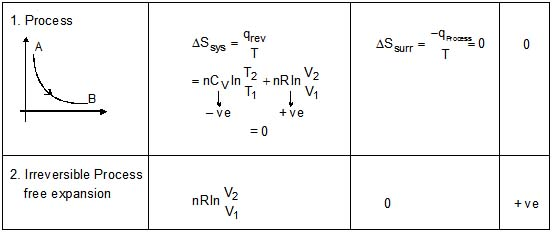For reversible process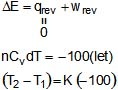Where K is constant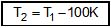For irreversible process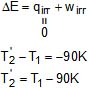This means that the final temperature of irreversible process is greater than reversible process.

ΔS = nCV ln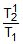+ nRln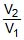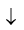-ve             +ve
= nCVln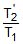- nCVln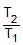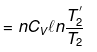⇒ T'2 > T2

⇒ ΔS = +ve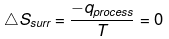⇒ ΔStotal = +ve

Calculation of entropy change in adiabatic compression.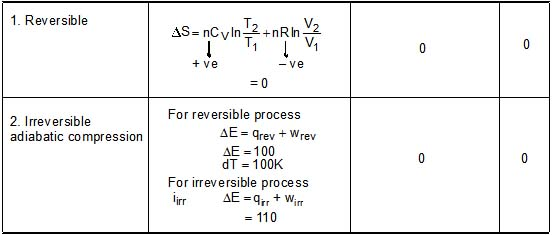dT = 110

For reversible

(T2 - T1) = 100K

T2 = T1 + 100 K

for irreversible
T'2= T1 110 K
T'> T2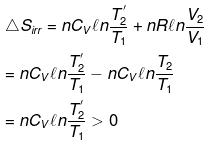Offer running on EduRev: Apply code STAYHOME200 to get INR 200 off on our premium plan EduRev Infinity!

## Chemistry for JEE

223 videos|452 docs|334 tests

,

,

,

,

,

,

,

,

,

,

,

,

,

,

,

,

,

,

,

,

,

,

,

,

;